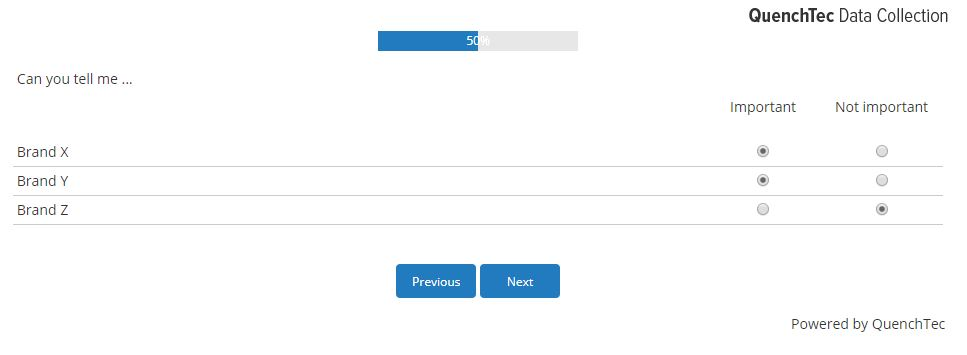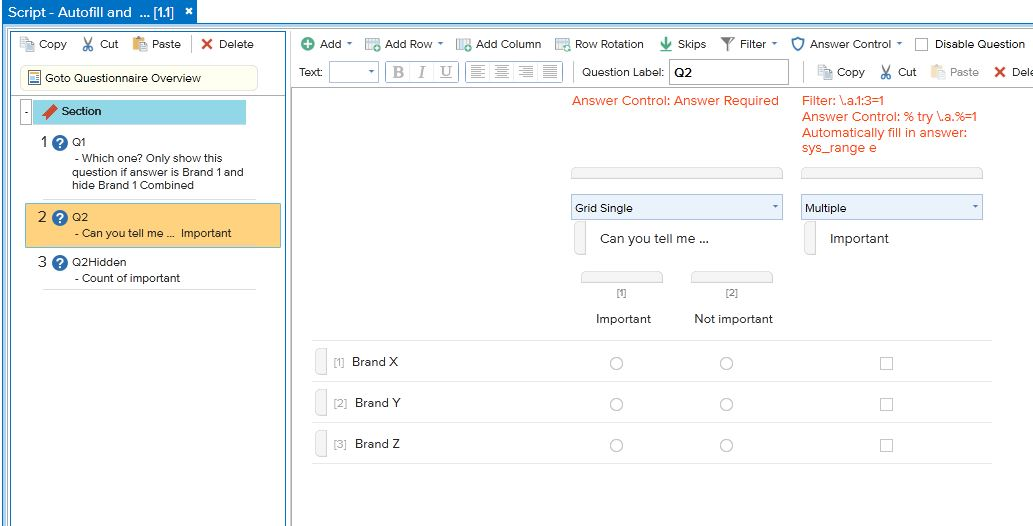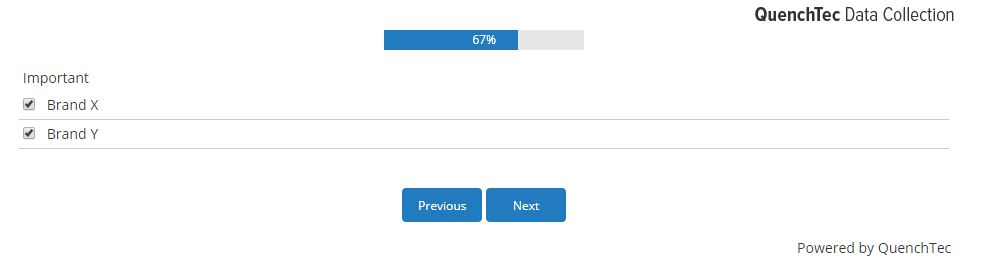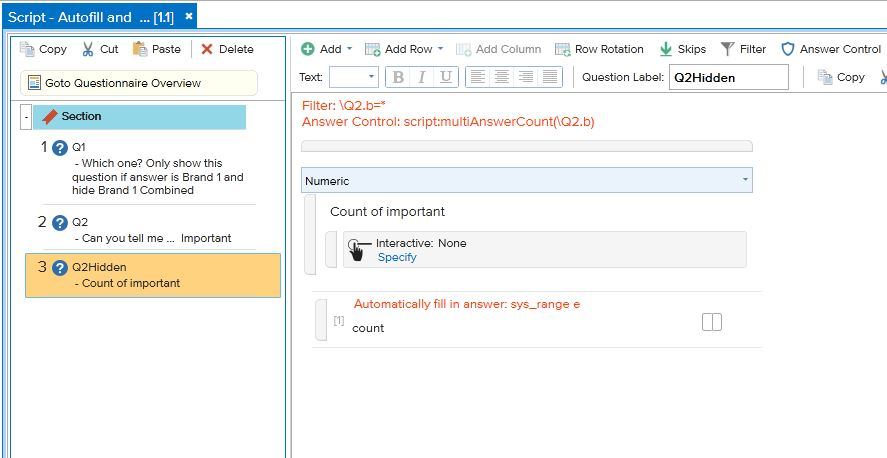For example we have a grid question with three rows and two columns.

We want to count how many people have answered column1.Create a subquestion where we autopunch the column1 answers.In here we have a filter: \.a.1:3=1

This means for subquestion 1 (a) for the rows 1 to 3 (1:3) the first response is answered (1)

In the Answer Contol we autopunch each of the selected row that has answer 1.

With sys_range e we can see show this question (with sys_range c you hide this question).Now we want to count the answers from this subquestion b.

Create a numeric question:In here we have a filter to get only the aswers selected from the subquestion from Q2 (this means Q2.b)